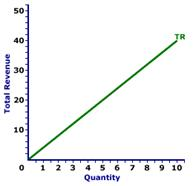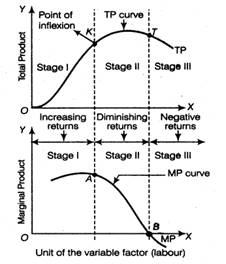### Production Function & Returns to a Factor - Test Papers

CBSE Test Paper-01

Class - 11 Economics (Producer’s Behaviour)

General Instruction:

• All questions are compulsory.
• Marks are given alongwith their questions.

1. With which of the following is the concept of marginal cost closely related? (1)
1. Variable cost
2. Fixed cost
3. Opportunity cost
4. Economic cost
2. Which of the following is true of the relationship between the marginal cost function and the average cost function? (1)
1. If MC curve is greater than ATC, then ATC is falling.
2. The ATC curve intersects the MC curve at minimum MC.
3. The MC curve intersects the ATC curve at minimum ATC.
4. If MC is less than ATC, then ATC is increasing.
3. What is the relationship between Total Revenue, Price, and Quantity sold by the firm related to each other? (2)
4. What do you understand by the term ‘Point of inflexion’? (2)
5. Production of gift pack one week before Diwali falls in which period? (3)
6. Do you agree with the view that TP increases even when MP is decreasing? (3)
7. ‘All expenses are production cost’. Do you agree? (4)
8. Is a producer in equilibrium under the following situations? Give reasons for your answer. (4)
1. When Marginal Revenue is greater than Marginal cost.
2. When Marginal Revenue is equal to Marginal cost.
9. From the following schedule, find out the level of output at which the producer is in equilibrium. Give reasons. (6)
 Output(units) Price(Rs.) Total cost(Rs.) 1 24 26 2 24 50 3 24 72 4 24 92 5 24 115 6 24 139 7 24 165
10. State giving reasons, whether the following statements are true or false.
1. When Total Revenue is constant, Average Revenue Will also be constant.
2. When Marginal Revenue falls to zero, Average Revenue Becomes maximum.
3. When Marginal Revenue is constant and not equal to zero then Total Revenue will also be constant.
4. When Marginal Revenue is zero, Average Revenue will be constant.
5. Marginal Revenue is always the price at which the last unit of the commodity is sold.
6. When Marginal Revenue is maximum, Marginal Revenue is also maximum.
7. When Marginal Revenue is positive and constant, Average Revenue and Total Revenue will both increase at a constant rate. (6)

CBSE Test Paper-01
Class–11 Economics (Producer’s Behaviour)

1. (a) Variable cost
2. (c) The MC curve intersects the ATC curve at minimum ATC.
3. Total revenue is considered as total money receipt of a producer on account of the sale of his total output. It is obtained by multiplying the per unit price of a commodity with the quantity of output sold.
TR = Price × Quantity
4. It is the point on a curve where the curve changes its slope, i.e. from increasing at an increasing rate to increasing at a diminishing rate and vice-versa.
5. Production of gift packs during Diwali season will fall under short period. As this period of Diwali is one week, so in this period, only variable factors like material, labour, etc. can be increased. Fixed factor like new plant and machinery cannot be increased. Hence, production period relates to due short-period.
6. Yes, TP increases even when MP is decreasing, because MP is an addition to TP. When MP is decreasing, only an addition to TP is decreasing i.e. TP continues to increase, though at a diminishing rate. TP starts declining only when MP becomes negative.
7. No, this is not true. Production cost refers to only those expenses which pertain to the production of goods. These costs may be direct (cost of material, labour, etc.) or indirect (electricity, telephone, etc.). the only condition being that they should be related to the production process either directly or indirectly. Hence, production cost includes the cost of factors of production.
1. No, if MR > MC, then it means that a producer is able to earn abnormal profits. This will motivate him to increase his production.
2. Yes, a producer will be in equilibrium where MR = MC and MC must be rising at this point. He will get maximum profit here.8.  Output(Q) (Units) Price(P) (Rs.) Total Cost (TC) (Rs.) Total Revenue(TR) (P × Q) (Rs.) Marginal Revenue(MR)(Rs.) (TRn – TRn-1) Marginal Cost(MC)(Rs.) (TCn – TCn-1) Profit(Rs.) (TR – TC) 1 24 26 24 24 26 -2 2 24 50 48 24 24 -2 3 24 72 72 24 22 0 4 24 92 96 24 20 4 5 24 115 120 24 23 5 6 24 139 144 24 24 5 7 24 165 168 24 26 3
The producer is in equilibrium at the 6th unit of output.
At an output level of 5th and 6th units, the difference between TR and TC (i.e. Profit) is maximum, which is equal to 5 in both the cases. But the producer is in equilibrium at 6th unit only where MR = MC and MC is rising.1. False, when total revenue is constant, average revenue will be diminishing, with the rise in output.
2. False, when marginal revenue is zero, average revenue will be diminishing but remains positive.
3. False, when marginal revenue is constant, total revenue will increase at a constant rate.
4. False, when marginal revenue is zero, average revenue should be diminishing but remains positive.
5. False, Marginal revenue is not always the price, at which the last unit of a commodity is sold. It simply refers to additional revenue, when an additional unit of output is sold, however when the price is constant, MR is equal to price.
6. False, when Total revenue is maximum, Marginal revenue is zero.
7. False, when marginal revenue is positive and constant, total revenue at a constant rate, but average revenue tends to be equal to marginal revenue.